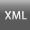Volume 9, Issue 1 (Special Issue on the 9th International Conference of Fuzzy Information and Engineering 2019)2019, 9(1): 19-29 Back to browse issues page
A new solving approach for fuzzy flexible programming problem in uncertainty conditions
F. Mahmoudi
Department of mathematics, University of Mazandaran, Babolsar, Iran
Abstract:   (1033 Views)
Modeling and solving real world problems is one of the most important issues in optimization problems. In this paper, we present an approach to solve Fuzzy Interval Flexible Linear Programming (FIFLP) problems that simultaneously have the interval ambiguity in the matrix of coefficients .In the first step, using the interval problem solving techniques; we transform the fuzzy interval flexible problem into two optimistic and pessimistic sub-problems. Then, in the second step, using a multi-parametric approach, we solve two fuzzy flexible sub- problems and finally the results are investigated with numerical example.

Keywords: Interval Linear Programming, Fuzzy Interval Flexible Linear Programming, –efficiency, –feasibility, Multi-parametric Approach